## Methods to measure the rate of reaction

• The rate of reaction can be measured in two ways:
(a) Average rate of reaction
(b) Rate of reaction at a given time
• The average rate of reaction is the average value of the rate of reaction within a specified period of time.
• Example: 0.2 g of a magnesium ribbon reacts completely with dilute hydrochloric acid in 40 seconds. What is the rate of reaction?
Solution: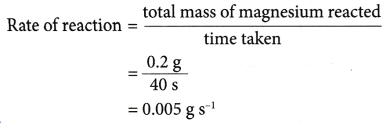• (a) The rate of reaction determined in above Example is known as the average rate of reaction.
(b) This is because it gives the average value of the rate of reaction within the 40 seconds.
• The average rate of reaction does not show the actual rate of reaction at a particular instant. Only the rate of reaction at a given time can be used to reflect the actual rate at that instant.
• Definition:
The rate of reaction at a given time is the actual rate of reaction at that instant.
The rate of reaction at a given time is also known as the instantaneous rate of reaction.

Calculating the rate of reaction for a reaction that liberates a gas:

For a reaction involving the liberation of a gas, the rate of reaction can be determined through the following ways.

• An experiment is carried out to measure the volume of gas collected at regular time intervals.
• A graph of the volume of gas collected against time is plotted.
• The graph plotted has the shape as shown in Figure.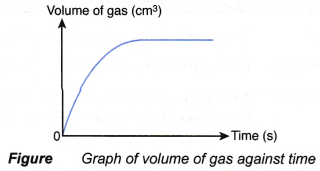• The graph can be used to determine the average rate of reaction and rate of reaction at a given time.

Determining the average rate of reaction from the graph: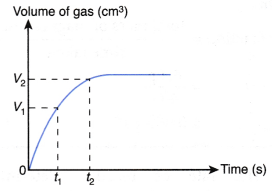From Figure,
the average rate of reaction in the first t1, second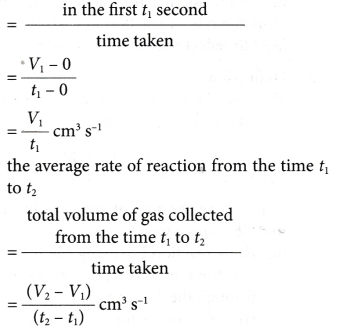Determining the rate of reaction at a given time from the graph:From Figure,
the gradient of the tangent at any point on the curve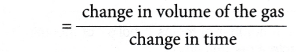This is the rate of reaction at that given time.
Hence,
Rate of reaction at a given time = gradient of the curve at that instant
The rate of reaction at a given time, t, can be calculated through the following steps.
Step 1: Draw a tangent (line AB) on the curve at the time t.
Step 2: Draw the right-angled triangle ABC.
Step 3: Measure the lengths of AC and BC.
Step 4: Calculate the gradient of the tangent AB.
Rate of reaction at time t
= gradient of the curve at time t
= gradient of the tangent ABShapes of curves using different selected quantities to measure the rate of reaction
Assuming a reaction is represented by the following equation:
R + T → W + X
The following changes occur as the reaction proceeds:

• Mass or concentration of the reactants R and T decreases with time.
• Mass or concentration of the products W and X increases with time.

For example, a reaction is carried out by mixing excess marble with dilute nitric acid. The chemical equation for the reaction is represented by the following equation:
CaCO3(s) + 2HNO3(aq) → Ca(NO3)2(aq) + CO2(g) + H2O(l)

If the concentration of nitric acid against time is plotted, the following graph will be obtained.The above graph is obtained based on the following facts:

• Concentration of nitric acid decreases with time.
• At the end of the reaction, all the nitric acid is completely reacted and its concentration becomes zero. This is due to the marble used in the reaction is in excess.

If the mass of marble against time is plotted, the following graph will be obtained.The above graph is obtained based on the following facts:

• Mass of marble decreases with time.
• At the end of the reaction, marble is in excess and m grams of marble remains unreacted.

If the concentration of calcium nitrate produced against time is plotted, the graph in Figure 1.8 is obtained.The above graph is obtained based on the following facts:

• As more and more calcium nitrate is produced, the concentration of calcium nitrate increases with time.
• When the reaction stops at time t, the concentration of calcium nitrate attains a maximum value, c mol dm-3.

If the volume of carbon dioxide gas produced against time is plotted, the following graph is obtained.The above graph is obtained based on the following facts:

• As more and more carbon dioxide gas is produced, volume of carbon dioxide gas increases with time.
• When the reaction stops at time t, the total volume of carbon dioxide gas achieves a maximum value, V cm3.

An experiment is carried out using the apparatus set-up as shown in Figure.Chemical equation for the reaction is
Zn(s) + H2SO4(aq) → ZnSO4(aq) + H2(g)
If the mass of the conical flask and its contents against time is plotted, the graph in Figure is obtained.The above graph is obtained based on the following facts:

• As the reaction proceeds, more and more hydrogen gas is produced. The gas escapes to the surroundings.
• Thus, the total mass of the conical flask and its contents decreases with time.
• When the reaction stops at time t, no more hydrogen gas is produced. Hence, the total mass of the conical flask and its contents achieves a minimum value, w g.

## How do you calculate the reaction rate experiment

Aim: To determine the average rate of reaction and the instantaneous rate of reaction.
Materials: Granulated zinc, 0.1 mol dm-3 hydrochloric acid, water.
Apparatus: 150 cm3 conical flask, burette, stopper fitted with a delivery tube, retort stand and clamp, stopwatch, basin, electronic balance, 50 cm3 measuring cylinder.
Procedure:

1. 25 cm3 of 0.1 mol dm-3 hydrochloric acid is measured and poured into a conical flask.
2. A burette is filled with water until it is full. It is then inverted over water in a basin and clamped vertically using a retort stand.
3. The water level in the burette is adjusted and the initial burette reading is recorded.
4. The apparatus as shown in Figure is set up.5. 5 g of granulated zinc is weighed using an electronic balance.
6. The granulated zinc is added into the conical flask.
7. The conical flask is closed immediately with a stopper fitted with a delivery tube as shown in Figure and the stopwatch is started at the same time.
8. The conical flask is shaken steadily throughout the experiment.
9. The volume of gas collected in the burette is recorded at intervals of 30 seconds for a period of 6 minutes.
10. The results are tabulated in a table.

Results:

 Time (s) 0 30 60 90 120 150 180 210 240 270 300 330 360 Burette reading (cm3) 48.9 41.4 37.15 34.4 32.4 30.65 29.15 27.9 27.15 26.4 25.65 25.15 24.9 Volume of gas liberated (cm3) 0 7.5 11.75 14.5 16.5 18.25 19.75 21 21.75 22.5 23.25 23.75 24

Interpreting data:

1. A graph of the volume of hydrogen gas liberated against time is plotted.2. To calculate the average rate of reaction3. To calculate the instantaneous rate of reaction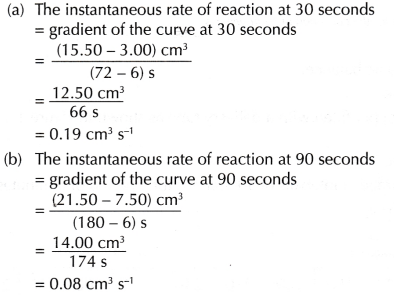Discussion:

• The instantaneous rate of reaction at 30 seconds (0.19 cm3 s-1) is higher than the instantaneous rate of reaction at 90 seconds (0.08 cm3 s-1).
The difference in rate is due to
(i) the concentration of hydrochloric acid at 90 seconds is lower than the concentration of hydrochloric acid at 30 seconds.
(ii) the total surface area of solid zinc at 90 seconds is smaller than the total surface area of solid zinc at 30 seconds.
• From the graph plotted, it can be seen that the gradient of the curve decreases with time. Hence, the rate of reaction decreases with time.
• As the reaction proceeds, the total surface area of zinc and the concentration of hydrochloric acid decrease with time. Hence, the rate of reaction also decreases with time. The rate of reaction will finally become zero, that is, the reaction stops when one or both the reactants are completely reacted.
The chemical equation for the reaction in this experiment is:
Zn(s) + 2HCl → ZnCl2(aq) + H2(g)
• Another method that can be used to measure the rate of reaction between zinc and hydrochloric acid is by measuring the change in mass of the conical flask and its contents against time.

Conclusion:
As a reaction proceeds, the rate of reaction decreases with time until it becomes zero, that is, the reaction finally stops.

## Rate of Reaction Calculation

Solving numerical problems
Examples about the calculation of the average rate of reaction and instantaneous rate of reaction are shown below.

1. 0.1 g of calcium carbonate is added to excess hydrochloric acid. The reaction stops after 15 seconds. 24.0 cm3 of carbon dioxide gas is collected. Calculate the average rate of reaction in
(a) g s-1
(b) mol s-1
(c) cm3 s-1.
[Relative atomic mass: C, 12; O, 16; Ca, 40]
Solution:2. In an experiment, one spatulaful of manganese(IV) oxide powder (as a catalyst) is added to 50 cm3 of sodium chlorate solution.The oxygen gas liberated is collected in a burette by downward displacement of water. Its volume is recorded at intervals of 2

 (s) 0 20 40 60 80 100 120 140 160 180 200 220 240 260 280 Volume of oxygen gas (cm3) 0 12 20.5 26.5 31.5 35.5 39 41.5 43.5 45.5 46.5 47.5 48.5 48.5 48.5

(a) Plot a graph of the volume of oxygen gas collected against time.
(b) Calculate the average rate of reaction
(i) in the first 2 minutes.
(ii) in the second minute.
(iii) for the overall reaction.
(c) Calculate the instantaneous rate of reaction
(i) at 20 seconds.
(ii) at 80 seconds.
Solution:
(a)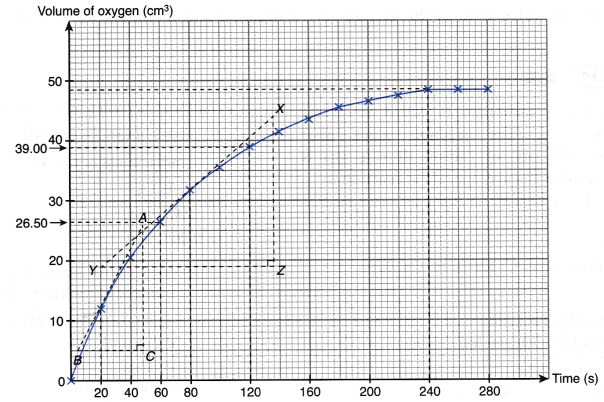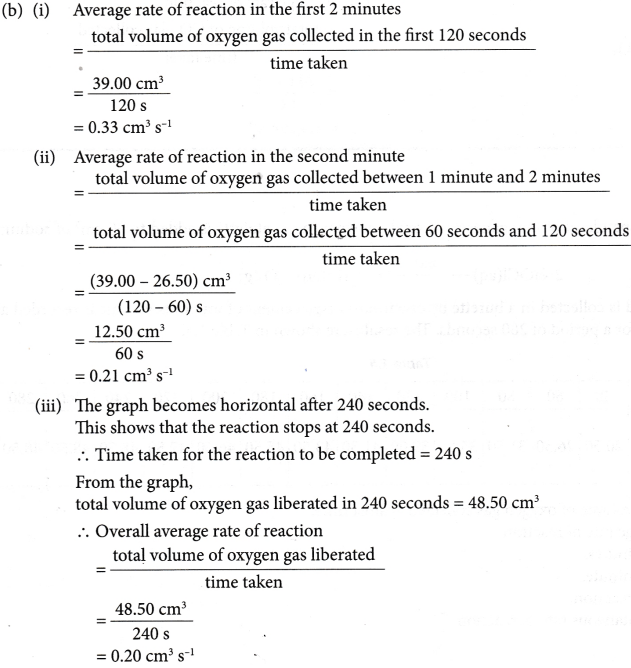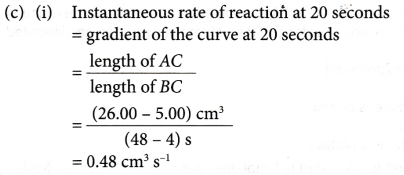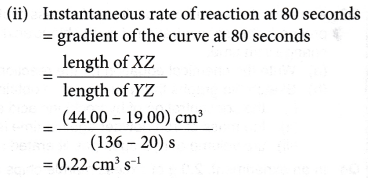0 seconds for a period of 280 seconds. The results are shown in Table.

 Time